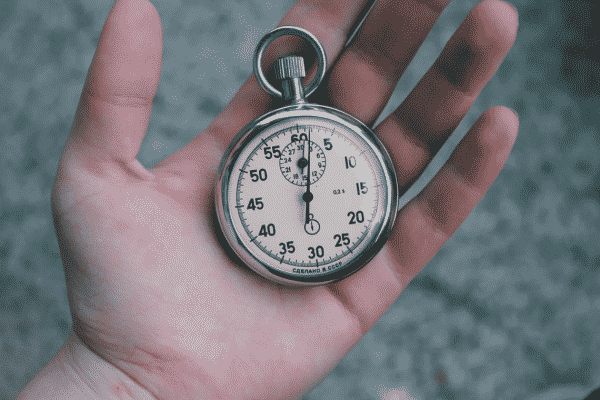# Speed, Distance and Time FAQ – How to Work out Speed Distance Time

Speed, distance and time questions are often used in technical positions whereby a person is required to carry out complicated calculations in a short space of time.

In this blog, I am going to cover some of the most frequently asked questions in relation to speed, distance and time. I have broken down this blog into questions, so that you can quickly find the questions you are looking for.

If there are any other questions you would like me to cover about the speed, distance and time tests, please leave a comment in the comments box below, and I will update this blog for you!## WHO USES SPEED, DISTANCE AND TIME TESTS?

Careers such as the Armed Forces often require people to undertake a speed distance and time test. The AOSB, OASC and AIB all use SDT tests to examine the potential of people who wish to join the Armed Forces.

It is, however, important to understand that this type of test is not limited to jobs in the Armed Forces. Other jobs in other fields may require you to sit a SDT test. Any job that requires strong technical ability will more than likely be asked to sit a SDT test as part of their initial application.## WHAT IS THE SPEED, DISTANCE TIME FORMULA?

There is a very simple formula to remember when it comes to working out speed, distance or time.

No matter what question you are asked, two of these variables (out of speed, distance and time) will ALWAYS be known. The one you don’t know is the one you are probably trying to work out.

The most effective way to remember how to calculate the speed, distance or time, is to use the following equation:## HOW DO YOU WORK OUT THE AVERAGE SPEED?

To work out the average speed, place your thumb over the speed variable (‘S’), and then do the equation.

Use this trick as a speed calculator to work out speed maths!

SPEED = DISTANCE ÷ TIMEEXAMPLE
What speed covers 34 miles in 10 minutes?

Step 1
• If we follow the advice in the previous method, we do not need to convert the hours into minutes, simply because it is already done for us. Therefore, speed = distance ÷ time

Step 2
• Speed = 34 ÷ 10

Step 3
• Calculate how many times does 10 go into 60 minutes? Answer = 6.
• Speed = 34 x 6 (6 x 10 = 60 minutes).

• 204 mph

## HOW DO YOU CALCULATE THE TIME?

To work out the time, place your thumb over the time variable (‘T’), and then do the equation.

TIME = DISTANCE ÷ SPEEDEXAMPLE
How long does it take to travel 48 miles at 20mph?

Step 1
• We know that the formula for calculating time = distance ÷ speed
• Time = 48/20

Step 2
• Following the same process as the method used to calculate speed, the denominator needs to go into 60 (minutes).
• In this case, 20 will go into 60 three times:
• Time = 48/20
• Time = 48 x 3 (3 x 20 = 60 minutes)

Step 3
• Time = 144 minutes

Step 4
• You now need to convert the ‘minutes’ into ‘hours and minutes’.

• 2 hours 24 minutes

## HOW DO YOU CALCULATE THE DISTANCE TRAVELLED?

To work out the distance travelled, place your thumb over the distance variable (‘D’), and then do the equation.

DISTANCE = SPEED X TIMEEXAMPLE
How far do you travel in 1 hour and 30 minutes at a constant speed of 40 mph?

Step 1
• We know that the formula for distance = speed x time.

Step 2
• We know that the distance = 40 x 1.5

• 60 miles

## DISTANCE-TIME GRAPHS

A distance-time graph can be used to represent the speed of an object. By drawing straight lines on the graph, you will be able to analyse the speed, distance and time of a moving object, and see whether or not that object is still.

The slope of the line represents acceleration. When an object is stationary, the straight line on the graph will be horizontal. The steeper the acceleration line, the faster the object is going.What can we see from this distance-time graph?

• In 1 hour, the object has travelled 15 miles. That means the object must be travelling at a speed of 15 mph (miles per hour).
• You can see that the object was stationary for 1 hour and 10 minutes (10 minutes on the first stop, and 1 hour on the second).
• The object took 1 hour to turn to its original position. That means the object’s speed was 19 miles per hour (because the object began at 19 miles and returned to its original position in exactly 1 hour).

## FURTHER RESOURCES

For more information on speed, distance and time questions, take a look at our SDT guide for a whole range of questions at a variety of difficulty levels.